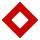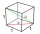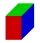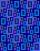# Diagonal + length - math problems

#### Number of problems found: 138

• Two diagonalsThe rhombus has a side length 12 cm and length of one diagonal 21 cm. What is the length of the second diagonal?
• DiamondSide length of diamond is 35 cm and the length of the diagonal is 56 cm. Calculate the height and length of the second diagonal.
• Find diagonalFind the length of the diagonal of a cuboid with length=20m width=25m height=150m
• Diagonals in diamons/rhombusRhombus ABCD has side length AB = 4 cm and a length of one diagonal of 6.4 cm. Calculate the length of the other diagonal.
• Rhombus diagonalArea of rhombus is 224. One diagonal measures 33, find length of other diagonal.
• RhombusCalculate the length of the diagonal AC of the rhombus ABCD, if its perimeter is 84 dm and the other diagonal BD has length 20 dm.
• Cube diagonalDetermine the length of the cube diagonal with edge 37 mm.
• Body diagonalCalculate the length of the body diagonal of the 6cm cube.
• Body diagonalFind the length of the body diagonal of a cuboid with lengths of 16 cm, 7 cm, and 4 cm.
• Body diagonalCalculate the cube volume, whose body diagonal size is 75 dm. Draw a picture and highlight the body diagonal.
• A squareA square with a length of diagonals 12cm give: a) Calculate the area of a square b) rhombus with the same area as the square, has one diagonal with a length of 16 cm. Calculate the length of the other diagonal.
• DiagonalCan a rhombus have the same length diagonal and side?
• RectangleThe rectangle has a perimeter 75 cm. Diagonal length is 32.5 cm. Determine the length of the sides.
• Body diagonalCuboid with base 7cm x 3,9cm and body diagonal 9cm long. Find the height of the cuboid and the length of the diagonal of the base,
• Diagonal 20Diagonal pathway for the rectangular town plaza whose length is 20 m longer than the width. if the pathway is 20 m shorter than twice the width. How long should the pathway be?
• Prism diagonalThe body diagonal of a regular square prism has an angle of 60 degrees with the base, the edge length is 10 cm. What is the volume of the prism?
• DiagonalDiagonal of the rectangle has length 46.4 cm. Angle between diagonal and londer sife of rectangle is 22°. Calculate area of the rectangle.
• A rhombusA rhombus has sides of length 10 cm, and the angle between two adjacent sides is 76 degrees. Find the length of the longer diagonal of the rhombus.
• Diagonals in the diamondThe length of one diagonal in diamond is 24 cm greater than the length of the second diagonal and diamond area is 50 m2. Determine the sizes of the diagonals.
• Diagonal to areaCalculate the area of a rectangle in which the length of the diagonal is 10 cm.

Do you have an interesting mathematical word problem that you can't solve it? Submit a math problem, and we can try to solve it.

We will send a solution to your e-mail address. Solved examples are also published here. Please enter the e-mail correctly and check whether you don't have a full mailbox.

Please do not submit problems from current active competitions such as Mathematical Olympiad, correspondence seminars etc...

Do you want to convert length units?